Get instant live expert help with Excel or Google Sheets“My Excelchat expert helped me in less than 20 minutes, saving me what would have been 5 hours of work!”

#### Post your problem and you’ll get expert help in seconds.

Your message must be at least 40 characters
Our professional experts are available now. Your privacy is guaranteed.

# How to find confidence interval in excel

We can apply the Descriptive Statistics tool to calculate confidence interval easily. Confidence interval specifies a range of values where there is a probability that the true value of a parameter lies. In this tutorial, we will learn the easy way of calculating confidence interval.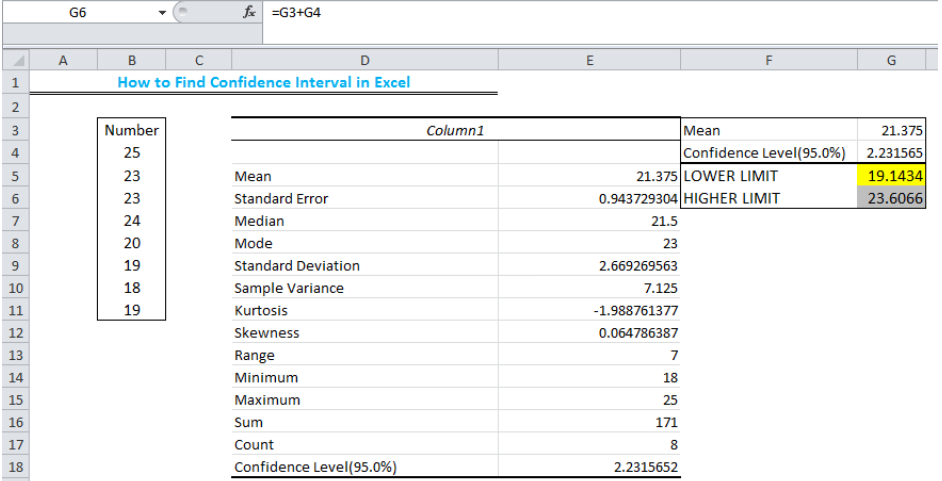Figure 1 – Result of 95% confidence interval

## How toFind 95% Confidence Interval

• We will use figure 2 to illustrate how we can find the 95% confidence interval of a set of values.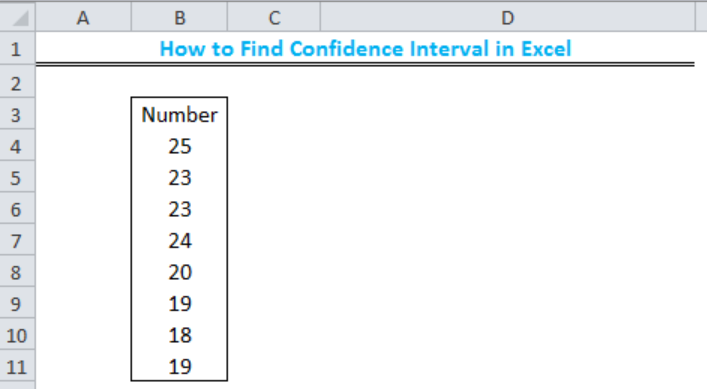Figure 2 – How to calculate confidence interval

• We will click the Data tab, and then, the Data Analysis tool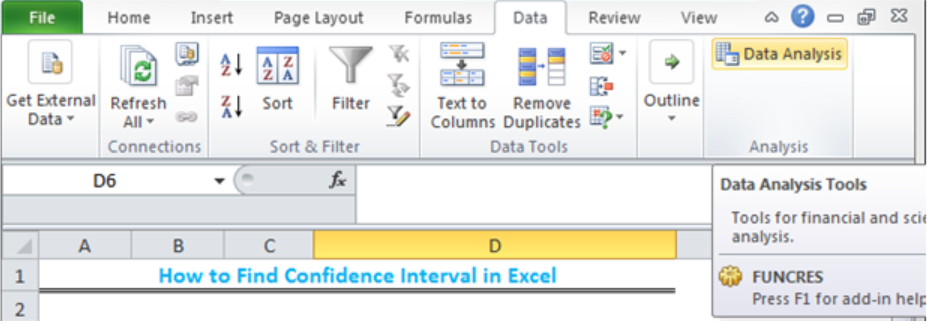Figure 3 – Click on Data Analysis

• If we do not have the Data Analysis tool, we should do the following:

• We will click File, Options, Add-Ins.
• We will click Go
• We will check the Analysis Toolpak box and the Analysis Toolpak VBA box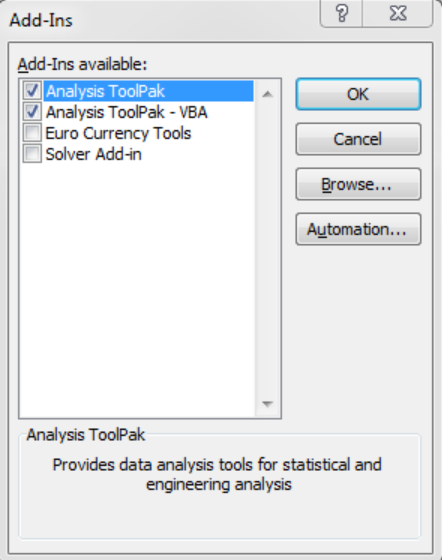Figure 4 – Inserting the Data Analysis tool

• Once we have clicked Data Analysis, we will see the Data Analysis dialog box. We will select Descriptive statistics and click OK
• On the descriptive statistics dialog box, we will click Input range and select the range of values from Cell B4 to Cell B11.  We will insert an Output range or check the New worksheet box for the result to be displayed in another worksheet. Our confidence interval will be set at 95% and we will check the Summary statistics box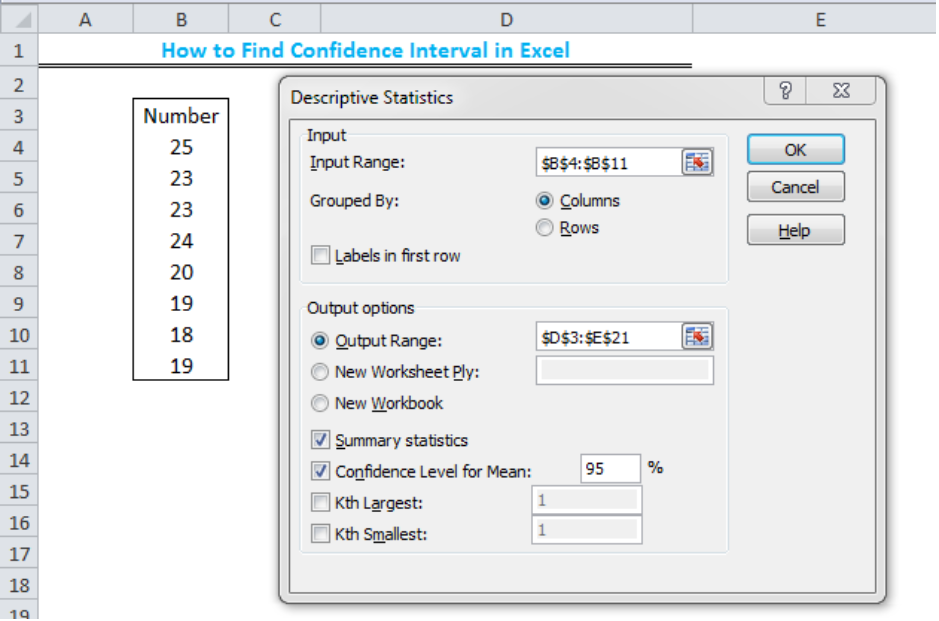Figure 5 – How to calculate 95% confidence interval

• We will click OK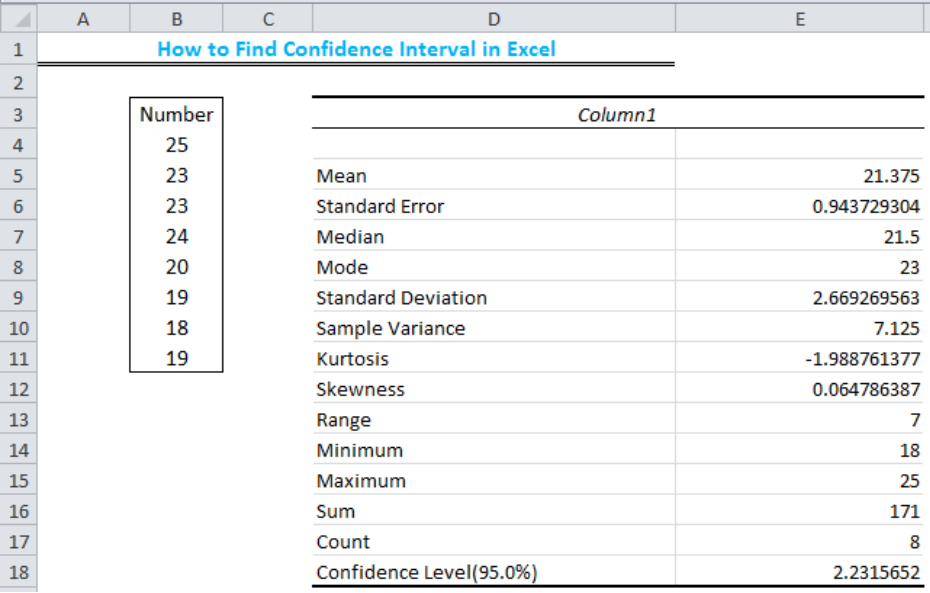Figure 6 – Result of Descriptive statistics

• Notice that in Row 18, the result is Confidence level and not Confidence interval
• To get the Confidence Interval, we need the Mean and the Confidence Level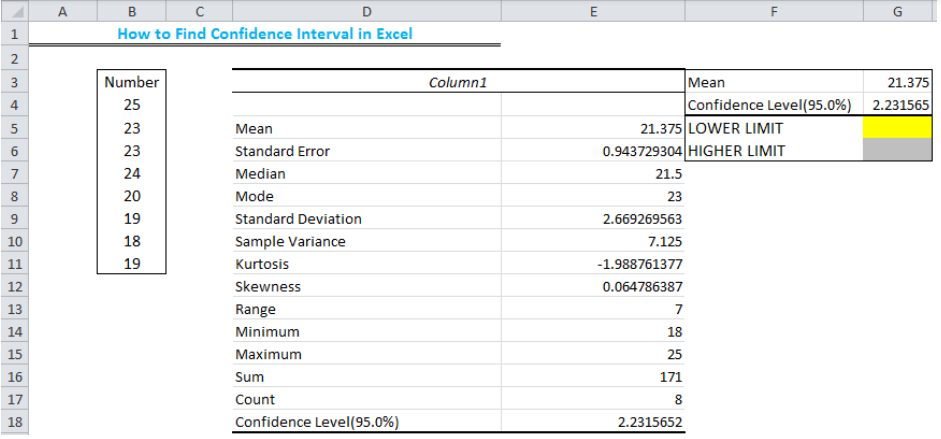Figure 7 – How to find the 95% confidence interval

• The Lower Limit is the mean minus the confidence level and the Higher limit is the mean plus the confidence level
• In Cell G5, we will paste the formula: =G3-G4
• In Cell G6, we will paste the formula: =G3+G4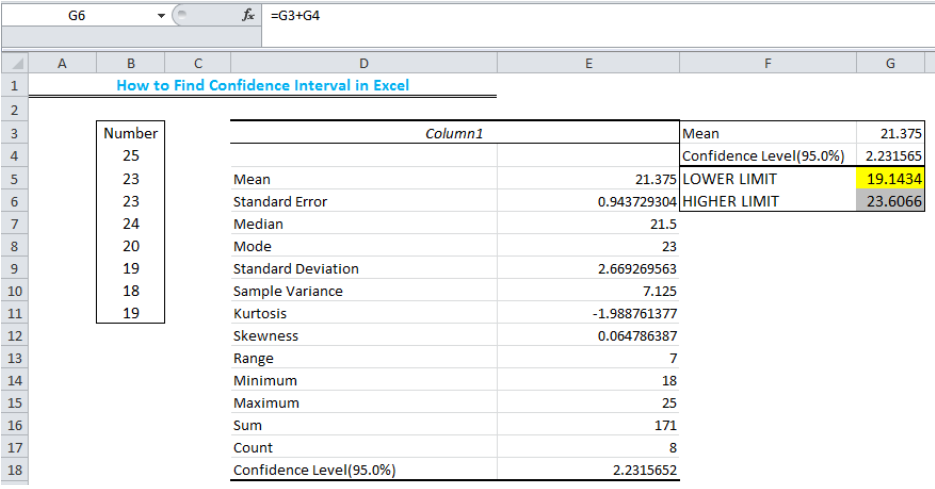Figure 8 – Result of 95% confidence interval

• The confidence interval of the values is 19.1434 ± 23.6066. To get the 90% Confidence Interval or any confidence level we want, we will simply adjust the Confidence level in Figure 5.

## Instant Connection to an Excel Expert

Most of the time, the problem you will need to solve will be more complex than a simple application of a formula or function. If you want to save hours of research and frustration, try our live Excelchat service! Our Excel Experts are available 24/7 to answer any Excel question you may have. We guarantee a connection within 30 seconds and a customized solution within 20 minutes.